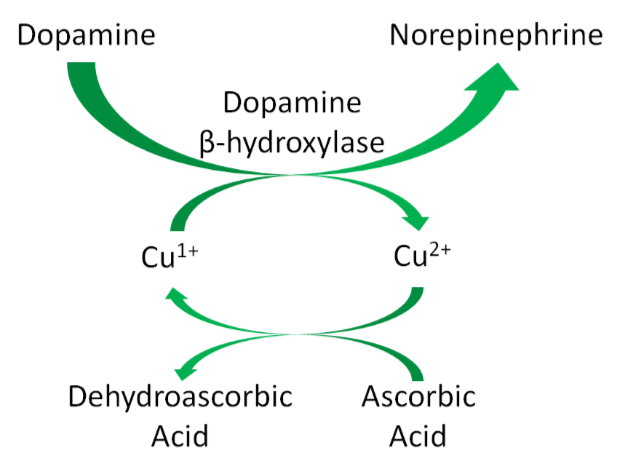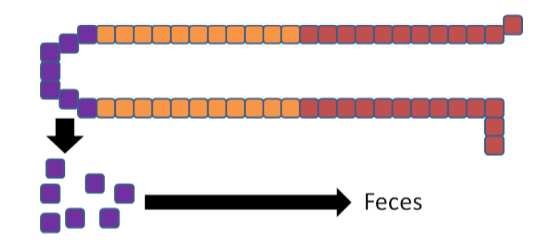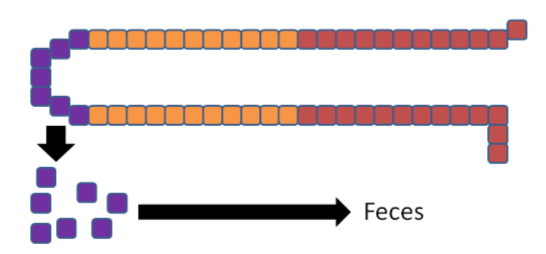Skip to main content

# 12.9: Copper

•• Contributed by Brian Lindshield
• Associate Prof (Department of Food, Nutrition, Dietetics and Health) at Kansas State University

Like iron, copper is found in 2 forms:

1. Cupric ($$\ce{Cu^2+}$$), oxidized
2. Cuprous ($$\ce{Cu^1+}$$), reduced

$$\ce{Cu^1+}$$ is the form that is primarily absorbed, thus $$\ce{Cu^2+}$$ is reduced to $$\ce{Cu^1+}$$ in the lumen. It is believed that, like iron, enzymatic reduction of $$\ce{Cu^2+}$$ is stimulated by ascorbate. The exact transporter that takes up the copper into the enterocyte is not known. It may be DMT1 that takes up non-heme iron. Once inside the enterocyte, $$\ce{Cu^1+}$$ can1:

1. Bind to thionein to form metallothionein. While zinc is a better stimulator of thionein levels, copper is actually a more avid binder to this protein.
2. Be used for functional uses as discussed in the next subsection.
3. Transported across the cell by an unknown carrier and then exported by ATP7A, an ATPase transporter.

Like zinc, copper is transported through the portal vein to the liver bound to albumin, as shown below. Albumin has a high affinity for $$\ce{Cu^2+}$$, so $$\ce{Cu^1+}$$ is oxidized before transported to albumin through ATP7A, as illustrated below.Figure $$\PageIndex{1}$$: Copper absorption

Similar to zinc, there is not much storage of copper in the body. The liver is the primary site of storage, where copper is taken up through an unknown transporter. If it is going to be stored, it will bind with thionein to form metallothionein. Copper is transported through circulation by the copper transport protein ceruloplasmin, which can bind 6 coppers/protein as shown below1.Figure $$\PageIndex{2}$$: Copper in the hepatocyte

Query $$\PageIndex{1}$$

Legumes, whole grains, nuts, shellfish, and seeds are good sources of copper2. It is estimated that over 50% of copper consumed is absorbed1. Copper is primarily excreted in the feces.

There are a number of different forms of copper used in supplements:

• Copper sulfate (25% copper)
• Cupric chloride (47% copper)
• Cupric acetate (35% copper)
• Copper carbonate (57% copper)
• Cupric oxide (80% copper)

All of these forms of copper are bioavailable, except cupric oxide. Assays have shown that it is not absorbed at all. Nevertheless, some supplements still use this form of copper1,3.

Query $$\PageIndex{2}$$

## Copper Functions

Copper has a number of functions that are described and shown below.

Two copper-containing proteins, ceruloplasmin and hephaestin, oxidize $$\ce{Fe^2+}$$ to $$\ce{Fe^3+}$$. $$\ce{Fe^3+}$$ is the form that binds to transferrin, as shown below1.Figure $$\PageIndex{3}$$: Transport and uptake of iron

Because copper is needed for this function, it is important for iron absorption.

Copper is also a cofactor for superoxide dismutase, which converts superoxide to hydrogen peroxide, as shown below.Figure $$\PageIndex{4}$$: Superoxide dismutase uses zinc as a cofactor

Copper is also needed for hormone synthesis. For example, it is a cofactor for dopamine beta-hydroxylase, which converts dopamine to norepinephrine, as shown below1.Figure $$\PageIndex{5}$$: Dopamine beta-hydroxylase requires copper4

Hopefully the following example looks vaguely familiar because we talked about this pathway in the vitamin C functions subsection. Ascorbic acid reduces $$\ce{Cu^2+}$$ back to $$\ce{Cu^1+}$$ so that this enzyme can continue to function, as shown below1. This is analogous to how ascorbic acid reduces $$\ce{Fe^3+}$$ back to $$\ce{Fe^2+}$$ so proline and lysyl hydroxylases can continue to function.Figure $$\PageIndex{6}$$: Dopamine beta-hydroxylase

Cytochrome c oxidase (complex IV) in the electron transport chain is a copper-containing enzyme that reduces oxygen to form water, as shown below1.Figure $$\PageIndex{7}$$: Cytochrome c oxidase (complex IV)5

Lysyl oxidase, an enzyme that is important for cross-linking between structural proteins (collagen and elastin), requires copper as a cofactor1.

Query $$\PageIndex{3}$$

## Copper Deficiency & Toxicity

Copper deficiency is rare in humans, but results in the following symptoms1,6:

• Hypochromic anemia
• Decreased white blood cell counts leading to decreased immune function
• Bone abnormalities

Copper deficiency can result in a secondary iron deficiency, since $$\ce{Fe^2+}$$ cannot be oxidized to $$\ce{Fe^3+}$$ to bind to transferrin. This can cause the hypochromic anemia that occurs in iron deficiency.

Menke's disease is a genetic disorder that results in copper deficiency. It is believed that individuals with this disease have a mutation in ATP7A that prevents copper from leaving the enterocyte, thus preventing absorption7.

Copper toxicity is also rare in humans, but acute toxicity results in the following symptoms1,6:

• Nausea, vomiting, diarrhea, abdominal pain

Chronic symptoms include1,6:

• Brain, liver, and kidney damage
• Neurological damage

Wilson's disease is a genetic disorder where a mutation in ATP7B prevents copper excretion, resulting in copper toxicity. One notable symptom is that individuals with this disease have golden to greenish-brown Kayser-Fleischer rings around the edges of the cornea, as shown in the link below1,6.Figure $$\PageIndex{8}$$: Kayser-Fleischer ring7

Query $$\PageIndex{4}$$

## How High Zinc Intake Can Lead to Copper & Iron Deficiencies

As you learned previously, thionein is the storage protein for zinc, but it more avidly binds copper. When it binds a mineral, it becomes metallothionein. High zinc intake results in increased thionein synthesis in the enterocyte. Thus, when an individual is consuming high zinc levels (most likely by supplementation, unlikely from dietary sources), the enterocyte will have high levels of thionein as shown below.Figure $$\PageIndex{9}$$: Zinc increases thionein production

The high levels of thionein will bind any copper that is taken up into the enterocyte (as metallothionein), "trapping" the copper in the enterocyte and preventing it from being absorbed into circulation, as shown below.Figure $$\PageIndex{10}$$: Copper taken up into the enterocyte is bound to thionein forming metallothionein.

The enterocytes containing the "trapped" copper move up the crypt and are sloughed off and excreted in feces. The copper consumed essentially is lost from the body through this process.Figure $$\PageIndex{11}$$: Enterocytes are sloughed off and excreted in feces

Without adequate copper being transported to the liver, no ceruloplasmin is produced and released into circulation. The lack of copper further influences iron transport by decreasing ceruloplasmin in circulation and hephaestin (another copper-containing protein) on the membrane of the enterocyte. These 2 proteins normally convert $$\ce{Fe^2+}$$ to $$\ce{Fe^3+}$$ so that iron can bind to transferrin.Figure $$\PageIndex{12}$$: Lack of copper means that hephaestin and ceruloplasmin aren't available to oxidize $$\ce{Fe^2+}$$ to $$\ce{Fe^3+}$$

Without hephaestin and ceruloplasmin, $$\ce{Fe^3+}$$ is not formed from $$\ce{Fe^2+}$$. As a result $$\ce{Fe^2+}$$ is "trapped" in the enterocyte because it can't bind to transferrin as shown below.Figure $$\PageIndex{13}$$: $$\ce{Fe^2+}$$ is trapped in the enterocyte

The enterocytes containing the "trapped" iron move up the crypt and are also sloughed off and excreted in feces. The iron consumed essentially is lost from the body through this process.Figure $$\PageIndex{14}$$: Enterocytes are sloughed off and excreted in feces

In summary, high zinc intake increases thionein production, which traps all copper; the lack of copper decreases circulating ceruloplasmin and hephaestin, which causes all iron to be trapped as well. This example illustrates the interconnectedness of zinc, copper, and iron.

Query $$\PageIndex{5}$$

## References

1. Gropper SS, Smith JL, Groff JL. (2008) Advanced nutrition and human metabolism. Belmont, CA: Wadsworth Publishing.
2. Whitney E, Rolfes SR. (2011) Understanding nutrition. Belmont, CA: Wadsworth Cengage Learning.
3. Baker DH. (1999) Cupric oxide should not be used as a copper supplement for either animals or humans. J Nutr 129(12): 2278-2279.
4. https://s3-us-west-2.amazonaws.com/c...467EB44654.png
5. http://wikidoc.org/index.php/File:ETC.PNG
6. Byrd-Bredbenner C, Moe G, Beshgetoor D, Berning J. (2009) Wardlaw's perspectives in nutrition. New York, NY: McGraw-Hill.
7. By Herbert L. Fred, MD, Hendrik A. van Dijk - http://cnx.org/content/m15007/latest/, CC BY 3.0, commons.wikimedia.org/w/inde...?curid=2746925
• Was this article helpful?